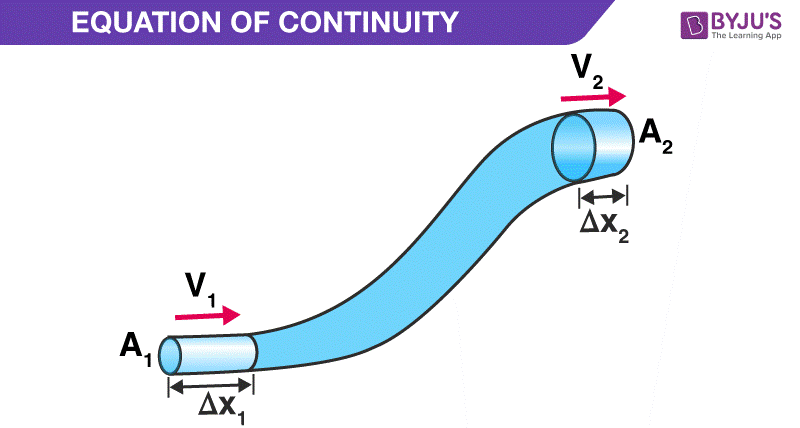Jet Set Go! All about Aeroplanes Jet Set Go! All about Aeroplanes

# Derivation of Continuity Equation

According to the continuity equation, the product of the cross-sectional area of the pipe and the velocity of the fluid at any given point along the pipe is constant.

In this session, we will learn about the derivation of the continuity equation.

## Continuity Equation DerivationContinuity equation represents that the product of cross-sectional area of the pipe and the fluid speed at any point along the pipe is always constant. This product is equal to the volume flow per second or simply the flow rate.  The continuity equation is given as:

 R = A v = constant

Where,

• R is the volume flow rate
• A is the flow area
• v is the flow velocity

### Assumption of Continuity Equation

Following are the assumptions of continuity equation:

• The tube is having a single entry and single exit
• The fluid flowing in the tube is non-viscous
• The flow is incompressible
• The fluid flow is steady

Related Article: Bernoulli’s Principle

### Derivation

Consider the following diagram:Now, consider the fluid flows for a short interval of time in the tube. So, assume that short interval of time as Δt. In this time, the fluid will cover a distance of Δx1 with a velocity v1 at the lower end of the pipe.

At this time, the distance covered by the fluid will be:

Δx1 = v1Δt

Now, at the lower end of the pipe, the volume of the fluid that will flow into the pipe will be:

V = A1 Δx1 = A1 v1 Δt

It is known that mass (m) = Density (ρ) × Volume (V). So, the mass of the fluid in Δx1 region will be:

Δm1= Density × Volume

=> Δm1 = ρ1A1v1Δt ——–(Equation 1)

Now, the mass flux has to be calculated at the lower end. Mass flux is simply defined as the mass of the fluid per unit time passing through any cross-sectional area. For the lower end with cross-sectional area A1, mass flux will be:

Δm1/Δt = ρ1A1v1 ——–(Equation 2)

Similarly, the mass flux at the upper end will be:

Δm2/Δt = ρ2A2v2 ——–(Equation 3)

Here, v2 is the velocity of the fluid through the upper end of the pipe i.e. through Δx2 , in Δt time and A2, is the cross-sectional area of the upper end.

In this, the density of the fluid between the lower end of the pipe and the upper end of the pipe remains the same with time as the flow is steady. So, the mass flux at the lower end of the pipe is equal to the mass flux at the upper end of the pipe i.e. Equation 2 = Equation 3.

Thus,

ρ1A1v1 = ρ2A2v2 ——–(Equation 4)

This can be written in a more general form as:

ρ A v = constant

The equation proves the law of conservation of mass in fluid dynamics. Also, if the fluid is incompressible, the density will remain constant for steady flow. So, ρ1 2.

Thus, Equation 4 can be now written as:

A1 v1 = A2 v2

This equation can be written in general form as:

A v = constant

Now, if R is the volume flow rate, the above equation can be expressed as:

R = A v = constant

This was the derivation of continuity equation.

## Watch the video and learn about the basics of continuity equation### Continuity Equation in Cylindrical Coordinates

Following is the continuity equation in cylindrical coordinates:

 $$\begin{array}{l}\frac{\partial \rho }{\partial t}+\frac{1}{r}\frac{\partial r\rho u}{\partial r}+\frac{1}{r}\frac{\partial \rho v}{\partial \theta }+\frac{\partial \rho w}{\partial z}=0\end{array}$$

### Incompressible Flow Continuity Equation

Following is the continuity equation for incompressible flow as the density, ρ = constant and is independent of space and time, we get:

 ∇.v = 0

Following is the continuity equation in cylindrical coordinates:

 $$\begin{array}{l}\frac{\partial }{\partial x}(\rho u)+\frac{\partial}{\partial y}(\rho v)+\frac{\partial }{\partial z}(\rho w)=0\end{array}$$

## Frequently Asked Questions – FAQs

### Define continuity equation.

According to the continuity equation, the product of the cross-sectional area of the pipe and the velocity of the fluid at any given point along the pipe is constant.

### Give the continuity equation.

R = A v = constant
Where,

• R is the volume flow rate
• A is the flow area
• v is the flow velocity

True.

∇.v = 0

### In which field the continuity equation is used?

The continuity equation is used in fluid dynamics.

Stay tuned with BYJU’S to learn more interesting science topics with engaging videos!

Test your knowledge on Continuity equation derivation